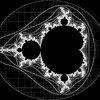## Dynamic Circle Chords II Black• Available
Products
57
• Artist
Notes

## Artist's Description

Code on Github

Based on Dynamic Circle Chords by Kyle Rollin

Created using Processing

int numRings = 1;
Ring[] rings = new Ring[numRings];

int numballs = 18;

void setup(){
size(8000,8000);
background(0);
stroke(255);
strokeWeight(15);
smooth();
ellipseMode(CENTER);
rings0 = new Ring(numballs, 4000, 4000, 3000);
noLoop();
}

void draw(){
background(0);
for (int i = 0; i < numRings; i++)
{
rings[i].changeN(numballs);
rings[i].display();
}
save("nodes_8015_"+numballs+".png");
exit();
}

void keyPressed(){
if (keyCode == UP)
{
numballs++;
}
if (keyCode == DOWN)
{
if (numballs > 1)
{
numballs—;
}
}
save("nodes_"+numballs+".tiff");
}

class Ring {
int numballs;
int xx, yy;

Ring(int n, int centx, int centy, int rad){
xx = centx;
yy = centy;
numballs = n;
}

void display(){
float degreeInc = 2.0*(PI / numballs);
int count = -1;
int[] pointsx = new int[numballs + 1];
int[] pointsy = new int[numballs + 1];
for ( float deg = 0; deg < 2*PI; deg = deg + degreeInc)
{
int dy = int(radius * sin(deg));
int dx = int(radius * cos(deg));
count++;
pointsx[count] = xx+dx;
pointsy[count] = yy+dy;
}

for (int i = 0; i <= count; i++)
{
for (int j = i+1; j <= count; j++)
{
line(pointsx[i],pointsy[i],pointsx[j],pointsy[j]);
}
}
}
void changeN(int n)
{
background(0);
numballs = n;

}

}